Updating search results...

# 13 Results

View
Selected filters:
• Common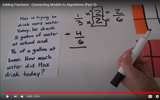Unrestricted Use
CC BY
Rating
0.0 stars
Overview:

VIDEO 3 OF 3 - This video addresses NC standard 5.NF.1. It explains how to connect the use of models for addition/subtraction of fractions to the standard algorithm for adding/subtracting fractions.

Subject:
Mathematics
Material Type:
Activity/Lab
Diagram/Illustration
Reference Material
Teaching/Learning Strategy
Author:
Dawne Coker
06/26/2020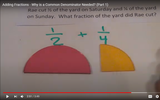Unrestricted Use
CC BY
Rating
0.0 stars
Overview:

VIDEO 1 OF 3 - This video addresses NC standard 5.NF.1. It explains WHY we create equivalent fractions when adding unlike denominators, and HOW to create equivalent fractions when adding unlike denominators.

Subject:
Mathematics
Material Type:
Demonstration
Diagram/Illustration
Reference Material
Author:
Dawne Coker
06/26/2020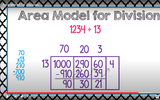Unrestricted Use
CC BY
Rating
0.0 stars
Overview:

This is one possible method for using the area model for division.

With this division strategy, students divide by breaking the dividend into its expanded form. Then, students use familiar multiplication facts to divide. It is suggested that this would be the first of three strategies for division (preceding partial quotients and the standard algorithm.

Subject:
Mathematics
Material Type:
Activity/Lab
Diagram/Illustration
Interactive
Teaching/Learning Strategy
Author:
Dawne Coker
06/28/2020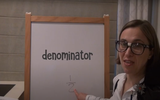Unrestricted Use
CC BY
Rating
0.0 stars
Overview:

This lesson is Day 10 in a series of 12 lessons around fraction equivalences and comparisons.

This video lesson serves as a review of equpartitioning in order to determine the fractional part of an area or length. This is a necessary foundational skill for comparing and finding equivalent fractions.

Subject:
Mathematics
Material Type:
Activity/Lab
Interactive
Lesson
Simulation
Author:
Dawne Coker
06/25/2020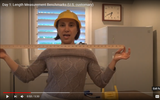Unrestricted Use
CC BY
Rating
0.0 stars
Overview:

This lesson is Day 1 in a series of 5 lessons around U.S customary measurement.

This 3rd grade video lesson introduces the U.S. customary measurements for length: inches, feet, and yards. In this lesson, students will learn benchmarks (visuals) for measuring in inches, feet and yards when they don't have standard measurement tools available.

Subject:
Mathematics
Material Type:
Activity/Lab
Interactive
Lesson
Simulation
Author:
Dawne Coker
06/25/2020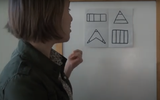Unrestricted Use
Public Domain
Rating
0.0 stars
Overview:

This lesson is Day 1 in a series of 12 lessons around fraction equivalences and comparisons.

This video lesson encourages students to reason about and visualize fractions. The lesson focuses on the ideas that fractions are fair shares and that fractions can be compared. This lesson assumes that students have some initial experiences with fractions.

Subject:
Mathematics
Material Type:
Interactive
Lesson
Presentation
Simulation
Author:
Dawne Coker
06/25/2020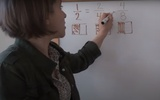Unrestricted Use
Public Domain
Rating
0.0 stars
Overview:

This lesson is Day 2 in a series of 12 lessons around fraction equivalences and comparisons.

This video lesson uses paper folding to introduce the concept of equivalent fractions. To complete this lesson, make sure you have a square piece of paper (any size) and a crayon

Subject:
Mathematics
Material Type:
Activity/Lab
Interactive
Lesson
Simulation
Author:
Dawne Coker
06/25/2020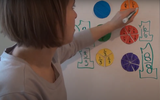Unrestricted Use
CC BY
Rating
0.0 stars
Overview:

This lesson is Day 2 in a series of 12 lessons around fraction equivalences and comparisons.

This video lesson introduces the idea that fractions can be used to represent 1 whole. To engage in this lesson, students may use virtual circle models from Toy Theater (https://toytheater.com/fraction-circles/)

Subject:
Mathematics
Material Type:
Activity/Lab
Interactive
Lesson
Simulation
Author:
Dawne Coker
06/25/2020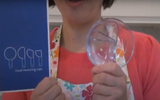Unrestricted Use
CC BY
Rating
0.0 stars
Overview:

This lesson is Day 4 in a series of 12 lessons around fraction equivalences and comparisons.

This video lesson looks at how we use fractions in the kitchen. Students are challenged to use circle models to find equivalent fractions for a recipe. Students may use virtual circe models from the Toy Theater (https://toytheater.com/fraction-circles/).

Subject:
Mathematics
Material Type:
Activity/Lab
Interactive
Lesson
Simulation
Author:
Dawne Coker
06/25/2020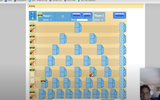Unrestricted Use
CC BY
Rating
0.0 stars
Overview:

This lesson is Day 2 in a series of 12 lessons around fraction equivalences and comparisons.

This game may be played with one or two people. It reviews fractions on a number line, adding fraction with like denominators, and equivalent fractions. For an extra challenge, you can even break up your moves across multiple tracks (e.g., if your card reads 1/2, you could move 1/4 and 2/8).

https://www.nctm.org/Classroom-Resources/Illuminations/Interactives/Playing-Fraction-Track/

Subject:
Mathematics
Material Type:
Activity/Lab
Interactive
Lesson
Simulation
Author:
Dawne Coker
06/25/2020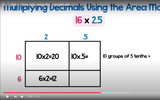Unrestricted Use
CC BY
Rating
0.0 stars
Overview:

The area model can be used when multiplying any two numbers, including decimals. This video demonstrates how to multiply using the area model when a decimal is involved.

Subject:
Mathematics
Material Type:
Activity/Lab
Diagram/Illustration
Interactive
Teaching/Learning Strategy
Author:
Dawne Coker
06/28/2020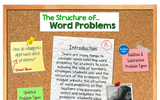Only Sharing Permitted
CC BY-ND
Rating
0.0 stars
Overview:

This Thinglink provides a series of brief videos addressing a) common areas of difficulty related to problem solving and b) an overview of additional and subtraction problem types.

Subject:
Mathematics
Material Type:
Activity/Lab
Diagram/Illustration
Interactive
Reference Material
Simulation
Author:
Dawne Coker
07/01/2020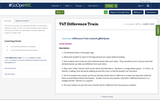Conditional Remix & Share Permitted
CC BY-NC-SA
Rating
3.0 stars
Overview:

This resource is part of Tools4NCTeachers.

In this lesson, students create a "train" (or row) of shapes. Each shape in the row must have one attribute in common with the shapes on either side.

Subject:
Mathematics
Material Type:
Lesson
Lesson Plan
Author:
DAWNE COKER